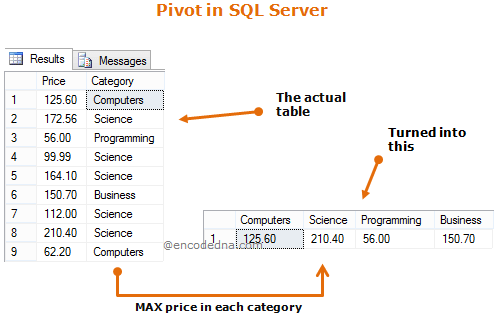﻿ Convert Rows to Columns in SQL Server using PIVOT

# Convert Rows to Columns in SQL Server using PIVOT

Ever wondered how you can convert data from rows to columns in SQL Server. I am talking about an SQL query, which will transform records from multiple rows into columns. Using SQL Server PIVOT, we can efficiently rotate a table’s data to show a summarized result.Usually, we PIVOT a table using unique values extracted from a column and show the result in multiple columns.

Let's see an example.

As usual, I'll use the dbo.Books table for the demo. The table has five columns and few rows in it. Create the table and insert the records in it.

Also, check this list of popular SQL Server post in this blog.

## PIVOT Using Aggregate function

I'll PIVOT (meaning, Turn) the Books table to get the max price for each distinct category. The T-SQL aggregate MAX() function will return the maximum value from a list of values in a column.

```SELECT Computers, Science, Programming, Business
FROM (
SELECT Price, Category FROM dbo.Books
) Books
PIVOT (
MAX(Price) FOR Category IN (Computers, Science, Programming, Business)
) Result;```

The output will look like,

`Computers 125.60Science210.40Programming56.00Business150.70`

Similarly, we can get the total price for each category using PIVOT and SUM() function.

```SELECT Computers, Science, Programming, Business
FROM (
SELECT Price, Category FROM dbo.Books
) Books
PIVOT (
SUM(Price) FOR Category IN (Computers, Science, Programming, Business)
) Result;```

### Output

`Computers 187.80Science759.05Programming56.00Business150.70`

The queries above have one similarity (other than using PIVOT), that it uses pre-defined values as column name (Computer, Science etc.). We looked for unique values in Category column and used the list in our query as columns.

Now, an obvious question that comes to our mind. How do we PIVOT a table if we do not know the values for our columns? It is not always wise to hardcore values as columns. Since, categories might change in the Books table or we add new categories to the list.

You may also like this post (a simple SQL trick): How to Insert multiple rows with a single INSERT statement in SQL Server?

## PIVOT using Dynamically extracted Columns

This may also be called as Dynamic PIVOT, where values for columns are extracted dynamically using an SQL query, at runtime.

We will first extract all the Distinct categories from the table and store the result in a variable. Each value is separate by a comma.

```DECLARE @Category AS VARCHAR(MAX)
SELECT @Category =
COALESCE(@Category + ', ', '') + CAST(Category AS VARCHAR(20))
FROM (SELECT DISTINCT Category FROM dbo.Books) Books
SELECT @Category Categories```

### Output

```Category
We got the values for our columns, all separate by a comma. Using the above result, we will now create the Dynamic PIVOT. The syntax remains the same as we did in Static PIVOT, except that we will add the variable @Columns inside the query and execute it as we execute a Stored Procedure.
```DECLARE @Category AS VARCHAR(MAX)
SELECT @Category =
COALESCE(@Category + ', ', '') + CAST(Category AS VARCHAR(20))
FROM (SELECT DISTINCT Category FROM dbo.Books) Books
DECLARE @DynamicPIVOT AS VARCHAR(MAX)
SELECT @DynamicPIVOT = 'SELECT ' + @Category +
' FROM (
SELECT Price, Category FROM dbo.Books
) Books
PIVOT (
MAX(Price) FOR Category IN (' + @Category + ')
) Result;'

EXEC (@DynamicPIVOT)```

### Output

`Business 150.70Computers125.60Programming56.00Science210.40`
Conclusion

You must use SQL Server 2005 and above to use PIVOT in your queries or procedures. SQL Server PIVOT simplifies the process of transforming data from rows to columns, where we use the row’s values as column headers. PIVOT usually works with aggregate functions, such as MAX(), SUM() etc.

Finally if you want, you can also save the PIVOT result in a Table.